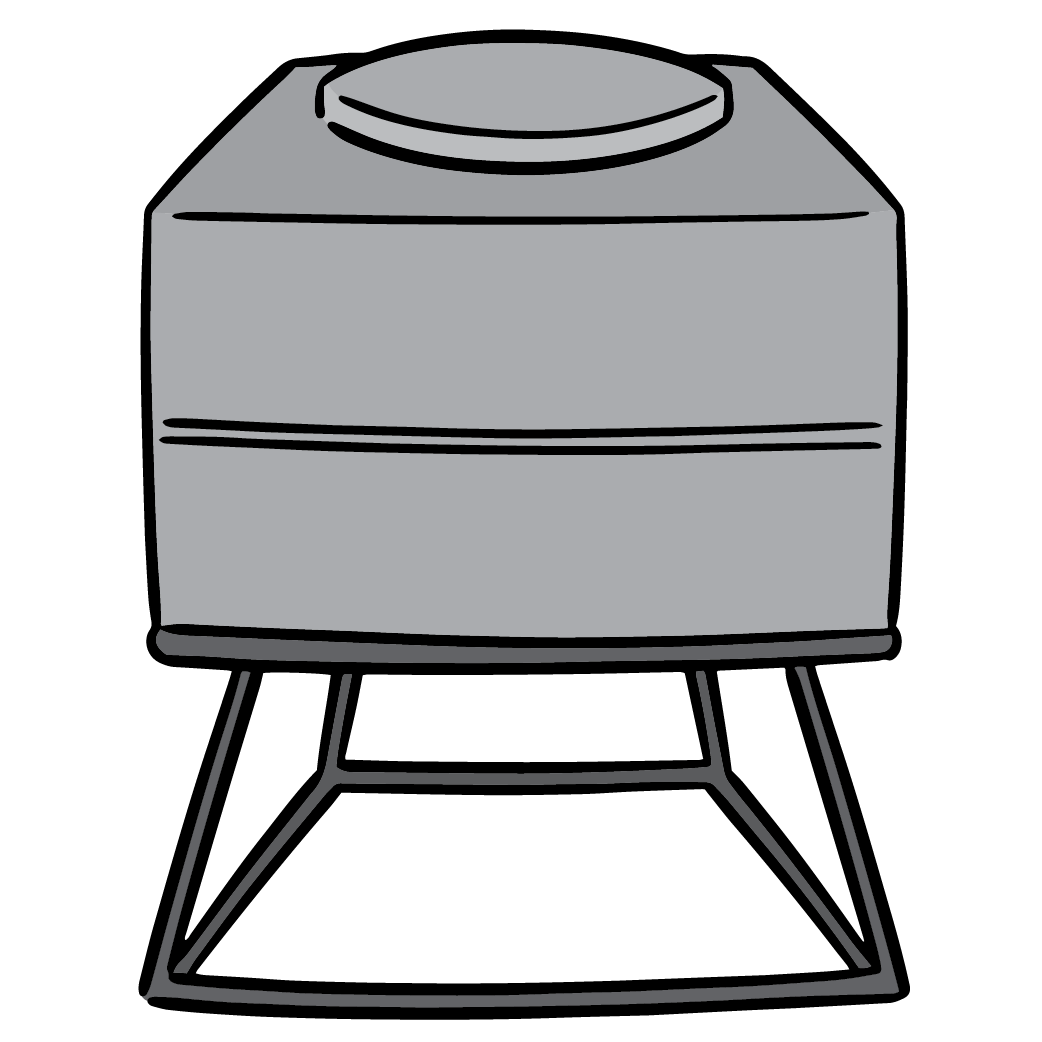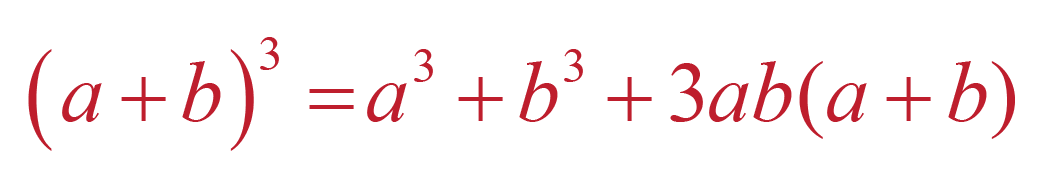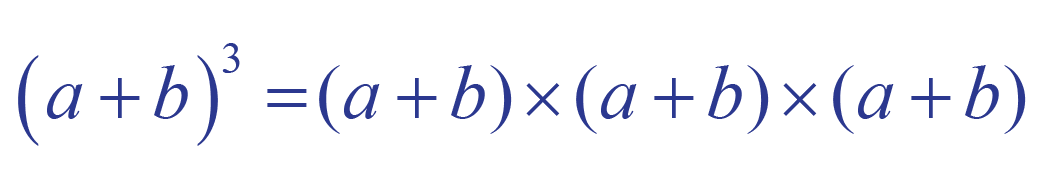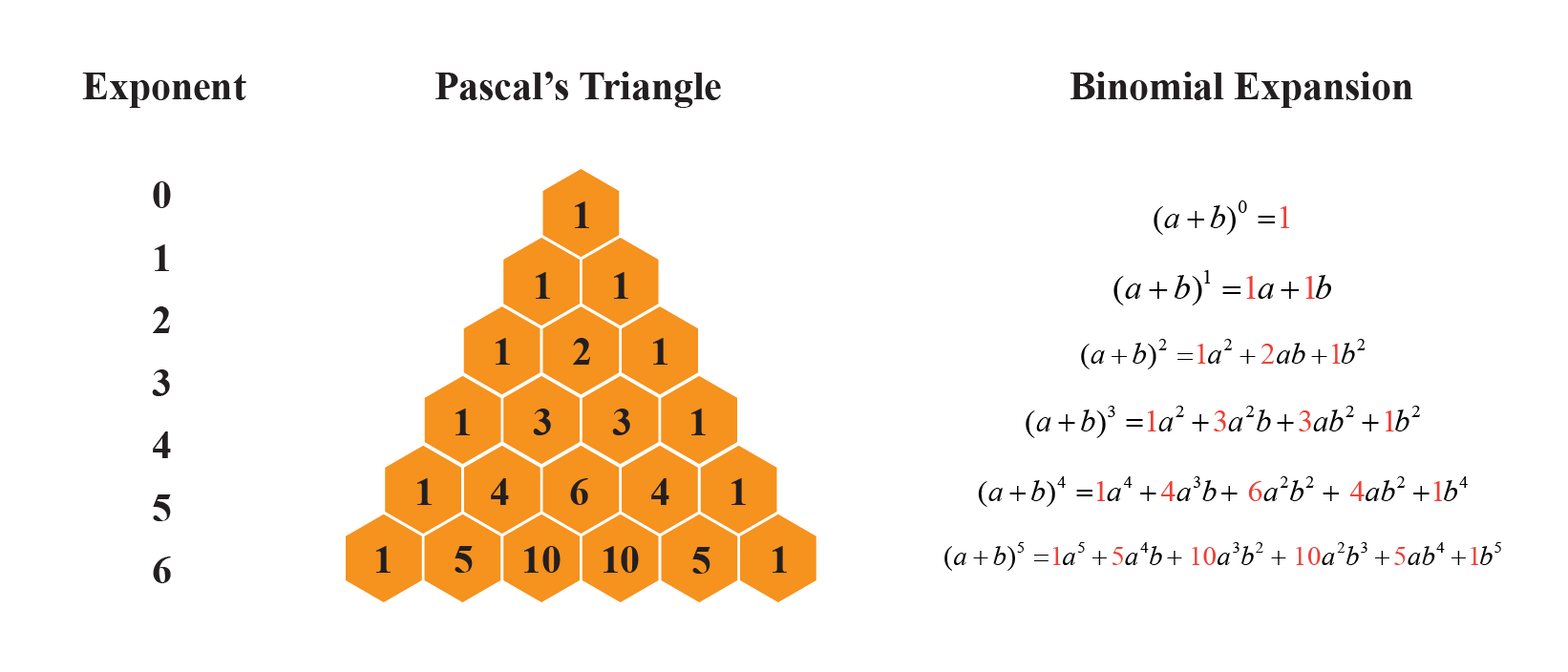# Cube of a Binomial

Cube of a Binomial

In this mini-lesson, we will learn about the cube of a binomial by understanding methods to find the cube of a binomial, and how to apply them while solving problems. We will also discover interesting facts around them.

John built a cubical water tank.

When his friend asked him what the length of the cube's side was. John replied it was  $$a + b$$.His friend then asked him how much water it could hold. John then suggested they cube the binomial, as the volume of a cube is $$l^3$$.

So, that volume of the cubical tank can be obtained by

${(a + b)}^3 = a^3 + b^3 +3ab(a + b)$The above equation is a cube of the binomial.

To write the cube of any number we simply multiply it three times to itself.

For example, cube of $$2$$ is $$2^3 = 2 \times 2 \times 2 = 8$$

Similarly, the cube of a binomial $$(a + b)$$ is

${(a + b)}^3 = (a + b) \times (a + b) \times (a + b)$Let's explore the cube of a binomial in more detail

## Lesson Plan

 1 How to Find the Cube of a Binomial? 2 Important Notes on Cube of a Binomial 3 Solved Examples on Cube of a Binomial 4 Challenging Questions on Cube of a Binomial 5 Interactive Questions on Cube of a Binomial

## How to Find the Cube of a Binomial?

Let's consider a basic binomial $${(x + y)}$$.

Cube of the above binomial is

 $${(x + y)}^3 = x^3 + y^3 + 3xy(x + y)$$

The above result is also an identity it holds true for every value of $$x$$ and $$y$$.

If we replace $$y$$ with $$-y$$ we can get.

 $${(x - y)}^3 = x^3 - y^3 - 3xy(x - y)$$

We can see that every $$+$$ sign in the expansion of $${(x + y)}^3$$ has changed to a $$-$$ sign in the expansion of $${(x - y)}^3$$

### Cubing a Binomial Steps

Let's see the steps to obtaining the above result for the binomial $${(x + y)}$$.

Step 1: First write the cube of the binomial $${(x + y)}^3 = (x + y) \times (x + y) \times (x + y)$$

Step 2: Multiply the first two binomials and keep the third one as it is

\begin{align} {(x + y)}^3 &= (x + y) \times (x + y) \times (x + y) \\[0.2cm] {(x + y)}^3 &= [x(x + y) + y(x + y)](x + y) \\[0.2cm] {(x + y)}^3 &= [x^2 + xy + xy + y^2](x + y) \\[0.2cm] {(x + y)}^3 &= [x^2 + 2xy + y^2](x + y) \end{align}

Step 3: Multiply the remaining binomial to the trinomial so obtained

\begin{align} {(x + y)}^3 &= [x^2 + 2xy + y^2](x + y) \\[0.2cm] {(x + y)}^3 &= x(x^2 + 2xy + y^2) + y(x^2 + 2xy + y^2) \\[0.2cm] {(x + y)}^3 &= x^3 + 2x^2y + xy^2 + x^2y + 2xy^2 + y^3 \\[0.2cm] {(x + y)}^3 &= x^3 + 2x^2y + x^2y + xy^2 + 2xy^2 + y^3 \\[0.2cm] {(x + y)}^3 &= x^3 + 3x^2y + 3xy^2 + y^3 \\[0.2cm] {(x + y)}^3 &= x^3 + y^3 + 3x^2y + 3xy^2 \\[0.2cm] {(x + y)}^3 &= x^3 + y^3 + 3xy(x + y) \end{align}

### Examples

Let's take a binomial in one variable $$(x + 1)$$.

The cube of the above binomial can be obtained following the above steps.

Step 1: First write the cube of the binomial $${(x + 1)}^3 = (x + 1) \times (x + 1) \times (x + 1)$$

Step 2: Multiply the first two binomials and keep the third one as it is.

\begin{align} {(x + 1)}^3 &= (x + 1) \times (x + 1) \times (x + 1) \\[0.2cm] {(x + 1)}^3 &= [x(x + 1) + 1(x + 1)](x + 1) \\[0.2cm] {(x + 1)}^3 &= [x^2 + x \times 1 + x \times 1 + 1^2](x + 1) \\[0.2cm] {(x + 1)}^3 &= [x^2 + 2x + 1](x + 1) \end{align}

Step 3: Multiply the remaining binomial to the trinomial so obtained.

\begin{align} {(x + 1)}^3 &= [x^2 + 2x + 1](x + 1) \\[0.2cm] {(x + 1)}^3 &= x(x^2 + 2x + 1) + 1(x^2 + 2x + 1) \\[0.2cm] {(x + 1)}^3 &= x^3 + 2x^2 + x + x^2 + 2x + 1 \\[0.2cm] {(x + 1)}^3 &= x^3 + 3x^2 + 3x + 1 \\[0.2cm] {(x + 1)}^3 &= x^3 + 1 + 3x(x + 1) \end{align}

Cube of the binomial $$(x - 1)$$ can now be written as

${(x - 1)}^3 = x^3 - 1 - 3x(x - 1)$

Explore the cube of a binomial calculator below to learn more about the steps of cubing a binomial.

## Solved Examples

 Example 1

Kevin cubed a binomial $$2x + 3y$$ and finds out that the coefficient of $$x^y$$ is 36, is he right?

Solution

Cube of the binomial $$2x + 3y$$ is $${(2x + 3y)}^3$$

We can use the identity $${(a + b)}^3 = a^3 + b^3 + 3ab(a + b)$$

Replace $$a$$ with $$2x$$ and $$b$$ with $$2y$$

\begin{align} {(2x + 3y)}^3 &= {(2x)}^3 + {(3y)}^3 + 3(2x)(3y)(2x + 3y) \\[0.2cm] {(2x + 3y)}^3 &= 8x^3 + 27y^3 + 18xy(2x + 3y) \\[0.2cm] {(2x + 3y)}^3 &= 8x^3 + 27y^3 + 18xy \times 2x + 18xy \times 3y \\[0.2cm] {(2x + 3y)}^3 &= 8x^3 + 27y^3 + 36x^y + 54xy^2 \end{align}

Coefficient of $$x^2y$$ is 36

 $$\therefore$$ Kevin is right
 Example 2

Mathew told to his friend that he can find the cube of the number $$999$$ without even multiplying it three times, explain how he finds the cube of $$999$$?

Solution

The number $$999$$ can be written as $$1000 - 1$$

$${(999)}^3 = {(1000 - 1)}^3$$

Using the identity $${(a - b)}^3 = a^3 - b^3 - 3ab(a - b)$$

Replace $$a$$ with $$1000$$ and $$b$$ with $$1$$

\begin{align} {(1000 - 1)}^3 &= {(1000)}^3 - 1^3 - 3(1000)(1)(1000 - 1) \\[0.2cm] {(1000 - 1)}^3 &= 1000000000 - 1 - 3(1000)(1)(999) \\[0.2cm] {(1000 - 1)}^3 &= 1000000000 - 1 - 2997000 \\[0.2cm] {(1000 - 1)}^3 &= 997002999 \end{align}

 $$\therefore$$ $${(999)}^3 = 997002999$$Challenging Questions
• Find $$a$$ if the coefficients of $$x^2$$ and $$x^3$$ in the expansion of $${(3+ax)}^9$$ are equal.

## Interactive Questions on Cube of a Binomial

Here are a few activities for you to practice.

## Let's Summarize

The mini-lesson targeted in the fascinating concept of the cube of a binomial. The math journey around the cube of binomial starts with what a student already knows, and goes on to creatively crafting a fresh concept in the young minds. Done in a way that not only it is relatable and easy to grasp, but also will stay with them forever. Here lies the magic with Cuemath.

At Cuemath, our team of math experts is dedicated to making learning fun for our favorite readers, the students!

Through an interactive and engaging learning-teaching-learning approach, the teachers explore all angles of a topic.

Be it worksheets, online classes, doubt sessions, or any other form of relation, it’s the logical thinking and smart learning approach that we at Cuemath believe in.

## FAQs on Cube of a Binomial

### 1. How do you simplify the cube of a binomial?

Cube of a binomial can be simplified using the identities:

$${(x + y)}^3 = x^3 + y^3 - 3xy(x + y)$$
$${(x - y)}^3 = x^3 - y^3 - 3xy(x - y)$$

### 2. What is a binomial?

A binomial is a type of polynomial that only has two terms.

For example, $$x + 2y$$, $$a^3 + 2b$$.

### 3. How do you expand a binomial?

A binomial can be expanded using the identities:

$${(x + y)}^3 = x^3 + y^3 - 3xy(x + y)$$
$${(x - y)}^3 = x^3 - y^3 - 3xy(x - y)$$

### 4. How do you expand a binomial using Pascal’s Triangle?

We can expand binomials using Pascal's triangle like:### 5. How do you cube expressions?

An expression can be cubed by multiplying itself three times.

For example, $${(a + b)}^3 = (a + b)(a + b)(a + b)$$

### 6. What are the steps for cubing a binomial?

Step 1: First write the cube of the binomial $${(x + y)}^3 = (x + y) \times (x + y) \times (x + y)$$.

Step 2: Multiply the first two binomials and keep the third one as it is.

Step 3: Multiply the remaining binomial to the trinomial so obtained.

### 7. What do you mean by the cube of a binomial?

A cube of a binomial is multiplying the binomial three times to itself.

### 8. How do you find the cube of a binomial?

A cube of a binomial can be found by multiplying to itself three times.

Or we can find the cube by using identity

$${(x + y)}^3 = x^3 + y^3 - 3xy(x + y)$$
$${(x - y)}^3 = x^3 - y^3 - 3xy(x - y)$$

Polynomials
Grade 9 | Questions Set 1
Polynomials
Grade 10 | Questions Set 1
Polynomials